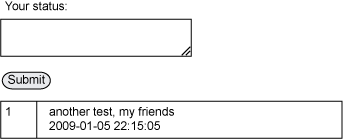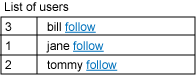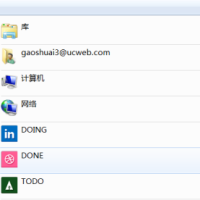## 使用 PHP 在站点上构建类似 Twitter 的系统 转

tweet（即贴子）存储在一个 posts 表中，每个贴子有一个主键（某种连续整数）、一个指向发出该贴的用户的外键关系、贴子本身（限制为一定数量的字符）和日期/时间戳。

 ``CREATE TABLE `users` (`id` INT NOT NULL AUTO_INCREMENT PRIMARY KEY ,`username` VARCHAR( 255 ) NOT NULL ,`email` VARCHAR( 255 ) NOT NULL ,`password` VARCHAR( 8 ) NOT NULL ,`status` ENUM( 'active', 'inactive' ) NOT NULL) ENGINE = MYISAM ;``

 ``CREATE TABLE `posts` (`id` INT NOT NULL AUTO_INCREMENT PRIMARY KEY ,`user_id` INT NOT NULL ,`body` VARCHAR( 140 ) NOT NULL ,`stamp` DATETIME NOT NULL) ENGINE = MYISAM ;``

 ``CREATE TABLE `following` (`user_id` INT NOT NULL ,`follower_id` INT NOT NULL ,PRIMARY KEY ( `user_id` , `follower_id` )) ENGINE = MYISAM ;``

 ``\$SERVER = 'localhost';\$USER = 'username';\$PASS = 'password';\$DATABASE = 'microblogger'; if (!(\$mylink = mysql_connect( \$SERVER, \$USER, \$PASS))){ echo "

Sorry, could not connect to database.1. 允许用户登录和添加贴子。
2. 将那些贴子转发给追随那个用户的人。
3. 允许用户追随其他用户。

 ``function add_post(\$userid,\$body){ \$sql = "insert into posts (user_id, body, stamp) values (\$userid, '". mysql_real_escape_string(\$body). "',now())"; \$result = mysql_query(\$sql);}``

 `` Microblogging Applicationvbody>". \$_SESSION['message'].""; unset(\$_SESSION['message']);}?>

``

 ``````function show_posts(\$userid){ \$posts = array(); \$sql = "select body, stamp from posts where user_id = '\$userid' order by stamp desc"; \$result = mysql_query(\$sql); while(\$data = mysql_fetch_object(\$result)){ \$posts[] = array( 'stamp' => \$data->stamp, 'userid' => \$userid, 'body' => \$data->body ); } return \$posts;}``

 `` \$list){ echo "
".\$list['userid'] ."".\$list['body'] ."
\n"; echo "".\$list['stamp'] ."
\n"; echo "\n"; echo "\n"; echo "\n";}?>

You haven't posted anything yet!

````function show_users(){ \$users = array(); \$sql = "select id, username from users where status='active' order by username"; \$result = mysql_query(\$sql); while (\$data = mysql_fetch_object(\$result)){ \$users[\$data->id] = \$data->username; } return \$users;}``

 `` Microblogging Application - Users

List of Users

\$value){ echo "
".\$key ."".\$value ." follow
\n"; echo "\n"; echo "\n"; echo "\n";}?>

There are no users in the system!

``

 ``

see list of users

````function following(\$userid){ \$users = array(); \$sql = "select distinct user_id from following where follower_id = '\$userid'"; \$result = mysql_query(\$sql); while(\$data = mysql_fetch_object(\$result)){ array_push(\$users, \$data->user_id); } return \$users;}``

 `` \$value){ echo "
".\$key ."".\$value; if (in_array(\$key,\$following)){ echo " unfollow "; }else{ echo " follow "; } echo "
\n"; echo "\n"; echo "\n"; echo "\n";}?>``

 ````

 ``function check_count(\$first, \$second){ \$sql = "select count(*) from following where user_id='\$second' and follower_id='\$first'"; \$result = mysql_query(\$sql); \$row = mysql_fetch_row(\$result); return \$row;}function follow_user(\$me,\$them){ \$count = check_count(\$me,\$them); if (\$count == 0){ \$sql = "insert into following (user_id, follower_id) values (\$them,\$me)"; \$result = mysql_query(\$sql); }}function unfollow_user(\$me,\$them){ \$count = check_count(\$me,\$them); if (\$count != 0){ \$sql = "delete from following where user_id='\$them' and follower_id='\$me' limit 1"; \$result = mysql_query(\$sql); }}``

 ``function show_users(\$user_id=0){ if (\$user_id > 0){ \$follow = array(); \$fsql = "select user_id from following where follower_id='\$user_id'"; \$fresult = mysql_query(\$fsql); while(\$f = mysql_fetch_object(\$fresult)){ array_push(\$follow, \$f->user_id); } if (count(\$follow)){ \$id_string = implode(',', \$follow); \$extra = " and id in (\$id_string)"; }else{ return array(); } } \$users = array(); \$sql = "select id, username from users where status='active' \$extra order by username"; \$result = mysql_query(\$sql); while (\$data = mysql_fetch_object(\$result)){ \$users[\$data->id] = \$data->username; } return \$users;}``

 ``

Users you're following

\$value){ echo "
• ".\$value."
• \n";}?>

You're not following anyone yet!

````function show_posts(\$userid,\$limit=0){ \$posts = array(); \$user_string = implode(',', \$userid); \$extra = " and id in (\$user_string)"; if (\$limit > 0){ \$extra = "limit \$limit"; }else{ \$extra = ''; } \$sql = "select user_id,body, stamp from posts where user_id in (\$user_string) order by stamp desc \$extra"; echo \$sql; \$result = mysql_query(\$sql); while(\$data = mysql_fetch_object(\$result)){ \$posts[] = array( 'stamp' => \$data->stamp, 'userid' => \$data->user_id, 'body' => \$data->body ); } return \$posts;}``

 ``\$users = show_users(\$_SESSION['userid']);if (count(\$users)){ \$myusers = array_keys(\$users);}else{ \$myusers = array();}\$myusers[] = \$_SESSION['userid'];\$posts = show_posts(\$myusers,5);``### SuShine

Netty那点事（三）Channel与Pipeline

Channel是理解和使用Netty的核心。Channel的涉及内容较多，这里我使用由浅入深的介绍方法。在这篇文章中，我们主要介绍Channel部分中Pipeline实现机制。为了避免枯燥，借用一下《盗梦空间》的...

2013/11/24
2W
22

jianglibo
2014/04/03
4.2K
3
CDH5: 使用parcels配置lzo

cloud-coder
2014/07/01
6.8K
1
5分钟 maven3 快速入门指南

fanl1982
2014/01/23
1.2W
7
DNS 管理系统--NamedManager

NamedManager 是一个基于 Web 的 DNS 管理系统，可用来添加、调整和删除 DNS 的 zones/records 数据，支持 Bind 作为后端的 DNS 服务，支持 IPv4 和 IPv6。...

2013/01/23
8.8K
0

28分钟前
0
0

osc_q5m9dzk0
28分钟前
19
0

0
0

BBuf

11
0

【前言】：公众号的AI初学者交流群已经建立啦，公众号后台回复【加群】可以加入。群里都是些一起学习的朋友，大家学习路上，结个伴。 【公众号资源汇总】：炼丹图书馆 | AI初学者所需要的资料...

0
0